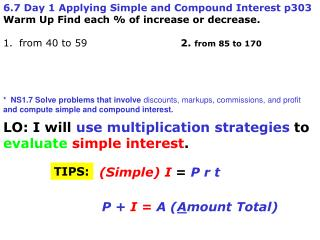DownloadDownload Presentation6.7 Day 1 Applying Simple and Compound Interest p303 Warm Up Find each % of increase or decrease.

# 6.7 Day 1 Applying Simple and Compound Interest p303 Warm Up Find each % of increase or decrease.

Télécharger la présentation## 6.7 Day 1 Applying Simple and Compound Interest p303 Warm Up Find each % of increase or decrease.

- - - - - - - - - - - - - - - - - - - - - - - - - - - E N D - - - - - - - - - - - - - - - - - - - - - - - - - - -
##### Presentation Transcript

1. 6.7 Day 1 Applying Simple and Compound Interest p303 • Warm Up Find each % of increase or decrease. • from 40 to 59 2. from 85 to 170 * NS1.7 Solve problems that involve discounts, markups,commissions, and profit and compute simple and compound interest. LO: I will use multiplicationstrategies to evaluatesimpleinterest. TIPS: (Simple) I= Prt P + I = A(Amount Total)

2. When you deposit money into a bank, the bank pays youinterest. • When you borrow money from a bank, you payinterest to the bank. Simple interestis money paid only on the principal. Rate of interest is the percent charged or earned. Time that the money is borrowed or invested (in years). Principalis the amount of money borrowedor invested. I= Prt

3. Finding Interest and Total Payment on a Loan-To buy a car, Jessica borrowed\$15,000 for 3 years at an annual simple interestrate of 9%.How much interest will she pay if she pays the entire loan off at the end of the third year? What is the total amount that she will repay? I = P rtUse the formula. First, find the interest she will pay. I = ______  ___  __ Substitute. ________ for 9%. Principal + Interest= Amount Total I = _____ _________ + __________ = _____________ Jessica will repay a total of \$__________ on her loan. Determining the Amount of Investment Time- Nancy invested \$6000 in a bond at a yearly rate of 3%. She earned \$450 in interest. Howlong was the money invested? I =P r tUse the formula. _____= _____  _____tSubstitute values _____ = ____t The money was invested for ______years, or ____ years and ____ months.

4. Computing Total Savings- John’s parents deposited \$1000 into a savings account as a college fund when he was born. How much will John have in this account after 18 years at a yearly simple interest rate of 4%? I = ____  ____  ____ I = ____ P + I = A The interest is \$____. Now find the total. ______ + _______ = A ______ = A John will have \$__________ in the account after 18 years. Finding the Rate of Interest- Mr. Twain borrowed \$8000 for 4 years to make home improvements. If he repaid a total of \$10,320, at what interest rate did he borrow the money? P + I= A (Amount Total) _____ + I = _______ He paid \$___ in interest. Now use the amount of interest paid to find the interest______ (%). ______= ______  r  ___ I = Prt _______= _______  r Divide both sides by 32,000. Mr. Twain borrowed the money at an annual rate of _____ or ___%. 6.7 RM & SRe p307

5. nt rn A= P(1 + ) 6.7 Day 2 Compound Interest p303 LO: I will evaluatecompound interestusing (Simple) I= Prt “and a little more.” Compound interest is INTEREST PAID on the PRINCIPAL, and ALSO on the INTEREST. A = final dollar value P = principal r = rate of interest t = time in years n = number of compounding periods per year.

6. nt rn A= P(1 + ) 2(12) 0.045t 2 = 1800(1 + ) David invested \$1800 in a savings account that pays 4.5% interest compounded semi-annually. Find the value of the investment in 12 years. Substitute. (Simple) I= Prt “and a little more.” = 1800(1 + 0.0225)24Simplify. = 1800(1.0225)24Add inside the parentheses. After 12 years, the investment will be worth about \$__,070.38. = ≈ 1800(1.70576)

7. Kia invested \$3700 in a savings account that pays 2.5% interest compounded quarterly. Find the value of the investment in 10 years. nt rn A= P(1 + ) _(__) 0.025t = 3700(1 + ) = 3700(1 + 0.00625)__Simplify. = 3700(1.00625)__ Add inside the parentheses. (Simple) I= Prt “and a little more.” ≈ 3700(1.28303) ≈ After 10 years, the investment will be worth about \$_______.

8. nt rn A= P(1 + ) The Hemmings borrowed \$3000 for home improvements. They repaid the loan and \$600 in simple interest four years later. What simple annual interest rate did they pay? Theresa invested \$800 in a savings account that pays 4% interest compounded quarterly. Find the value of the investment after 6 years. (Simple) I= Prt “and a little more.” (Simple) I= Prt “and a little more.” RTGO? Quiz Page 308 all